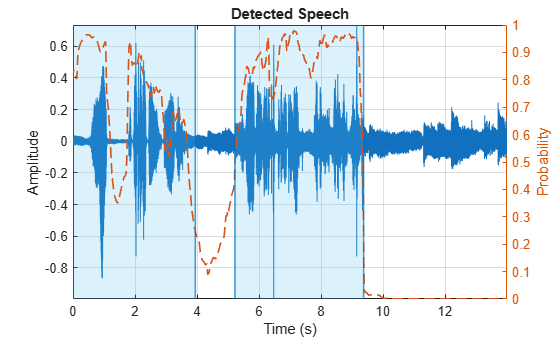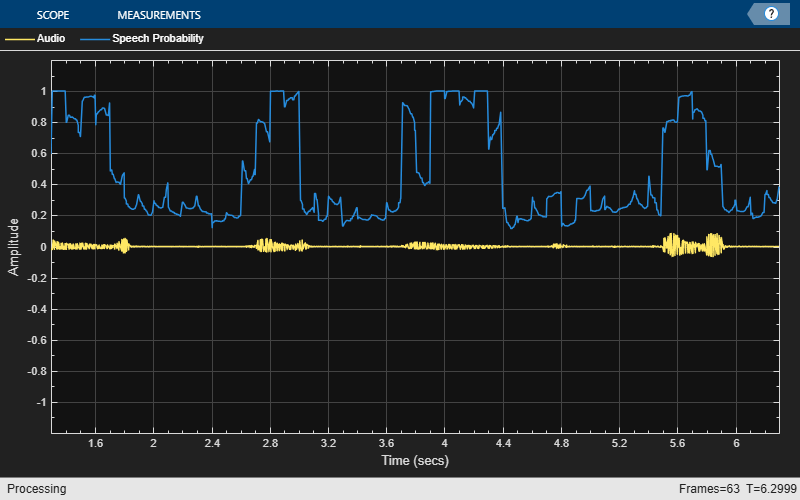Preprocess audio for voice activity detection (VAD) network

Since R2023a

## Syntax

``features = vadnetPreprocess(audioIn,fs)``

## Description

example

````features = vadnetPreprocess(audioIn,fs)` returns a mel spectrogram from the audio input that you can feed to the pretrained network returned by `vadnet`.```

## Examples

collapse all

Read in an audio signal containing speech and music and listen to the sound.

```[audioIn,fs] = audioread("MusicAndSpeech-16-mono-14secs.ogg"); sound(audioIn,fs)```

Use `vadnetPreprocess` to preprocess the audio by computing a mel spectrogram.

`features = vadnetPreprocess(audioIn,fs);`

Call `vadnet` to obtain a pretrained VAD neural network.

`net = vadnet;`

Pass the preprocessed audio through the network to obtain the probability of speech in each frame.

`probs = predict(net,features);`

Use `vadnetPosprocess` to postprocess the network output and determine the boundaries of the speech regions in the signal.

`roi = vadnetPostprocess(audioIn,fs,probs)`
```roi = 2×2 1 63120 83600 150000 ```

Plot the audio with the detected speech regions.

`vadnetPostprocess(audioIn,fs,probs)`Create a `dsp.AudioFileReader` object to stream an audio file for processing. Set the `SamplesPerFrame` property to read 100 ms nonoverlapping chunks from the signal.

```afr = dsp.AudioFileReader("MaleVolumeUp-16-mono-6secs.ogg"); analysisDuration = 0.1; % seconds afr.SamplesPerFrame = floor(analysisDuration*afr.SampleRate);```

The `vadnet` architecture does not retain state between calls, and it performs best when analyzing larger chunks of audio signals. When you use `vadnet` in a streaming scenario, specific application requirements of accuracy, computational efficiency, and latency dictate the analysis duration and whether to overlap analysis chunks.

Create a `timescope` object to plot the audio signal and the corresponding speech probabilities. Create an `audioDeviceWriter` to play the audio as you stream it.

```scope = timescope(NumInputPorts=2, ... SampleRate=afr.SampleRate, ... TimeSpanSource="property",TimeSpan=5, ... YLimits=[-1.2,1.2], ... ShowLegend=true,ChannelNames=["Audio","Speech Probability"]); adw = audioDeviceWriter(afr.SampleRate);```

Call `vadnet` to obtain a pretrained VAD neural network.

`net = vadnet();`

In a streaming loop:

1. Read in a 100 ms chunk from the audio file.

2. Preprocess the audio into a mel spectrogram using `vadnetPreprocess`.

3. Use the VAD network to predict the probability of speech in each frame of the spectrogram. Replicate the probabilities to correspond to each sample in the audio signal.

4. Plot the audio signal and the probabilities of speech.

5. Play the audio with the device writer.

```hop = 0.01 * afr.SampleRate; while ~isDone(afr) audioIn = afr(); features = vadnetPreprocess(audioIn,afr.SampleRate); probs = predict(net,features); % Replicate probs to correspond to samples in audioIn probs = repelem(probs,hop)'; probs = probs((hop/2)+1:end-hop/2); scope(audioIn,probs) adw(audioIn); end```## Input Arguments

collapse all

Audio input signal, specified as a column vector (single channel).

Data Types: `single` | `double`

Sample rate in Hz, specified as a positive scalar.

Data Types: `single` | `double`

## Output Arguments

collapse all

Mel spectrogram, returned as a 40-by-T matrix, where T is the number of spectra in the spectrogram.

## Algorithms

The `vadnetPreprocess` function preprocesses the audio data using the following steps.

1. Resample the audio to 16kHz.

2. Compute a centered short-time Fourier transform (STFT) using a 25 ms periodic Hamming window and 10 ms hop length. Pad the signal so that the first window is centered at 0 s.

3. Convert the STFT to a power spectrogram.

4. Apply a mel filter bank with 40 bands to obtain a mel spectrogram.

5. Convert the mel spectrogram to a log scale.

6. Standardize each of the mel bands to have zero mean and standard deviation of 1.

## Version History

Introduced in R2023a